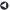### Statistical Services OfferedBack to List of Services

#### Power Analysis

The power of a hypothesis test is the probability of rejecting the null hypothesis (H0) when the alternative hypothesis (H1) is true.* Power and sample size analysis optimizes the resource usage and design of a study, improving chances of conclusive results with maximum efficiency. If the sample size is too small, it may not be possible to demonstrate significant results for a study. This may necessitate additional expenditures to re-do the study with a larger sample size. Conversely, if the sample size is excessive, valuable resources may have been wasted because significant results could have been demonstrated with a smaller sample size.

(*Note: Another more general definition of power is the probability of rejecting H0 for any given set of circumstances, even those corresponding to H0 being true. The SAS POWER procedure uses this more general definition.)

Two new procedures, PROC POWER and PROC GLMPOWER, for power analysis became available with SAS/STAT software (SAS 9.1).

The POWER procedure covers power analysis for the following:

• t tests, equivalence tests, and confidence intervals for means
• tests, equivalence tests, and confidence intervals for binomial proportions
• multiple regression
• tests of correlation and partial correlation
• one-way analysis of variance
• rank tests for comparing two survival curves
• logistic regression with binary response
• Wilcoxon Mann-Whitney rank-sum test

The GLMPOWER procedure covers power analysis for Type III (Partial) tests and contrasts of fixed effects in univariate linear models, optionally with covariates. The covariates can be continuous or categorical. Test and contrasts involving random effects are not supported.